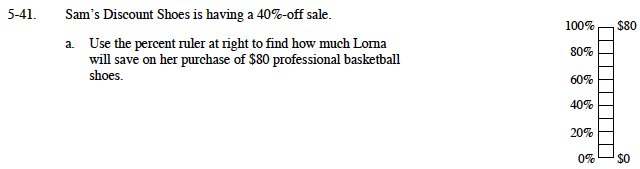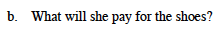### Home > MC1 > Chapter 5 > Lesson 5.2.1 > Problem5-41

5-41.First, you can scale the right side of the percent ruler with increments in dollars.

Because there are 10 spaces between the values of $0 and$80, the scaling increments will be eighty divided by ten. Can you find the increments and complete the scale? This way you can find the dollar equivalent of 40%.The discount of 40% means that the dollar amount will be taken away from the total amount. Using your answer from part (a), subtract her discount from the original price to find the amount Lorna will actually pay.

(Original Price) − (40% Discount) = New Price

Lorna will pay \$48.